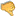# Uncertainty = Infinite Probabilities ÷ EnergyHassan Ajami
2021 / 7 / 24

The equation u = infinite Ps ÷ E is a law of nature, such that u is uncertainty, infinite Ps is all of the infinite probabilities, and E is energy. If there are different and opposing probabilities, then and only then there is uncertainty. This is why we are justified in analyzing uncertainty in terms of probabilities. In light of this consideration, the maximum uncertainty is equivalent to the maximum number of probabilities which amounts to infinite probabilities. This leads to the conclusion that the highest degree of uncertainty should be analyzed in terms of infinite probabilities, exactly as the previous law says.

The law u = infinite Ps ÷ E is a special case of the general law u = the sum of all the Ps ÷ E, when the sum of all the Ps (i.e. probabilities) is infinite. We could call this law the uncertainability law because it describes how uncertainty implies the existence of certain abilities due to implying probabilities and energy. Now, we need energy, i.e. E, in this law in order to enable the probabilities to be manifested because without energy nothing could be done. The law u = the sum of all the Ps ÷ E accounts for this fact in the following way: since u = the sum of all the Ps ÷ E, it follows that E × u = the sum of all the Ps, leading to the conclusion that more energy is needed to obtain more probabilities. The success of the uncertainability law in accounting for the fact that more energy is needed in order to obtain more probabilities speaks for the acceptance of this law.

From the perspective of the dominant theory in modern physics, the energy of the universe is equal to zero because the positive energy of the universe is equal to its negative energy. Now, since u = infinite Ps ÷ E, and given that the energy of the universe is equal to zero, it follows that u is indefinite, because any number divided by zero (such as the infinite Ps divided by zero) is indefinite. This conclusion that u is indefinite means that uncertainty is indefinite, which is logical and consistent. All of this shows that the law u = infinite Ps ÷ E is consistent because it entails a consistent result, namely that uncertainty is nothing but being indefinite.

If the law u = the sum of all the Ps ÷ E is true, then more energy entails less uncertainty, and less energy entails more uncertainty. Therefore, the law u = the sum of all the Ps ÷ E predicts that the basic building blocks of nature have the least possible energy, otherwise the universe wouldn’t have been governed by uncertainty, such as the uncertainty principle of Heisenberg. From this perspective, if the bit of information is equivalent to the minimum possible energy, then the universe is constructed out of information as some physicists say, such as John Wheeler. This shows that the law u = the sum of all the Ps ÷ E is compatible with the scientific paradigm which analyzes the universe as being a set of information.

According to the law u = the sum of all the Ps ÷ E, uncertainty is the measure of probabilities and energy. So even if we start with uncertainty (such as nothingness governed by uncertainty), we will end up with the existence of probabilities and energy, leading to the formation of universes, such as our own universe.Bad 12345678910 Very good